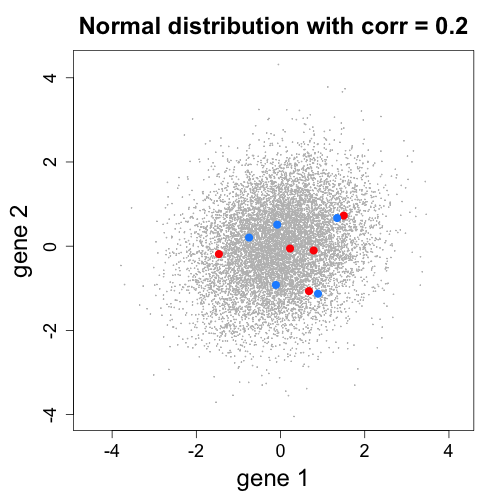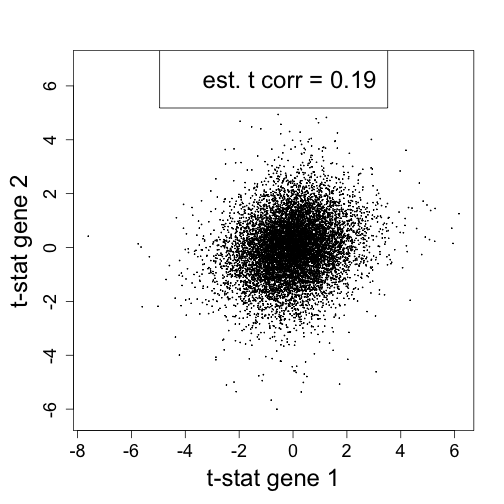# Gene set testing and correlations

Michael Love
June 8, 2015

### Gene set tests with correlation

Consider the average t-statistic from $$N$$ genes in a set $$G$$:

$\bar{t}=\frac{1}{N} \sum_{i \in G} t_i$

This statistic $$\bar{t}$$ combines the information about DE from the set and might be a useful test statistic.

### Gene set tests with correlation

Under the null hypothesis, the $$t$$ have mean 0. If the $$t$$ are independent then $$\sqrt{N} \bar{t}$$ has standard deviation 1 and is approximately normal:

$\sqrt{N} \bar{t} \sim N(0,1)$

This comes from the following decomposition of the variance:

$\begin{eqnarray} \text{Var}(\bar{t}) &=& \frac{1}{N^2} \text{Var}(t_1 + \dots + t_N) \\ &=& \frac{1}{N^2}( \text{Var}(t_1) + \dots + \text{Var}(t_N) ) \\ &=& \frac{1}{N} \end{eqnarray}$

### Gene set tests with correlation

Now consider the case that the test statistics $$t_i$$ in a gene set are not independent but have correlation $$\rho$$ under the null hypothesis.

$\bar{t}=\frac{1}{N} \sum_{i \in G} t_i$

$\text{corr}(t_i, t_{i'}) = \rho, \quad i, i' \in G$

The variance of the average t-statistics will be:

$\begin{eqnarray} \text{Var}(\bar{t}) &=& \frac{1}{N^2} \text{Var}( (1 \dots 1) (t_1 \dots t_N)' ) \\ &=& \frac{1}{N^2}(1 \dots 1) \begin{pmatrix} 1 & \rho & \dots & \rho & \rho \\ \rho & 1 & \rho & \dots & \rho \\ \dots & \dots & \dots & \dots & \dots \\ \rho & \rho & \dots & \rho & 1 \\ \end{pmatrix} (1 \dots 1) ' \\ &=& \frac{1}{N^2}\{N + (N-1) N \rho \} \\ &=& \frac{1}{N}\{1 + (N-1) \rho \} \end{eqnarray}$

### Variance inflation with correlation

So the variance inflation factor (VIF) comparing the independent case to the case with correlation is:

$VIF = 1 + (N-1) \bar{\rho}$

So the increased width (standard deviation) of the null distribution for a gene set with 20 genes and average correlation 0.1 will be:

sqrt(1 + 19 * 0.1)

 1.702939


This VIF is approximately true also for testing the set statistics against the complement: the genes not in the set (see Barry, Nobel and Wright 2008).

### Test statistic vs expression correlations

Here, the expression of 5 samples vs 5 samples, no true difference but a correlation of gene expression.### Test statistic vs expression correlations

If the test statistic $$T$$ is a linear form of the data $$X$$ (e.g. log fold change), then:

$\rho^T_{i,i'} = \rho^X_{i,i'}$

For t-test, the relationship is monotone, approximately linear and:

$\rho^T_{i,i'} \approx \rho^X_{i,i'}$

(Barry, Nobel and Wright, 2008)

### Simulate expression correlation of 0.2